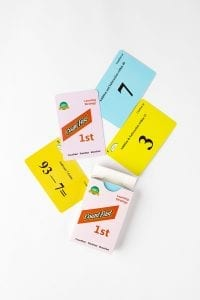# CountFast 1st Home Activities

####OVERVIEW & PURPOSE

Week 1 of the 3rd Grade CountFast program focuses on the review of quickly adding and subtracting smaller numbers within 120. Spend 15 minutes each day on one of the activities listed in this module.  Card decks should go home with students each day for additional practice with a parent at home. Each week, a new deck is introduced, and the previous deck is for the student to keep at home for continued practice.

#### EDUCATION STANDARDS

1. Math.Content.2.NBT.B.5
Fluently add and subtract within 100 using strategies based on place value, properties of operations, and/or the relationship between addition and subtraction.
2. NCTM Standard: develop a sense of whole numbers and represent and use them in flexible ways, including relating, composing, and decomposing numbers

#### OBJECTIVES

1. Mentally add and subtract within 120.
2. Develop fluency with mental addition and subtraction calculations.
DAY 1:Use the yellow cards from the deck.  These have the numbers 1 – 5, along with header cards of 15+ and 15-.  Have your child add up the numbers 1-5.  They should end up at 15.  (Ex.  1 + 2 = 3, 3 + 3 = 6, 6 + 4 = 10, 10 + 5 = 15).  You child should be able to show you that he/she can mix up the cards one through five and still add up to 15 every time.  Repeat this activity, but this time subtract the numbers 1-5 from 15.   (Ex. 15 – 5 = 10, 10 – 4 = 6, 6 – 3 = 3, 3 – 2 = 1, 1 – 1 = 0).  Again, your child should be able to demonstrate that the cards one through five can be subtracted from 15 in any order, and still end up at zero.  There are two cards that you can use for keeping track of how fast your child can add up to or subtract down from 15.  As you child becomes more confident with the process, challenge him/her to add up or subtract down as quickly as possible.  Record the time on the cards and encourage your child to bead his/her time each round of practice.

DAY 2:

Use the same procedure as yesterday.  This time, your child will use the blue cards in the deck to show that adding the numbers 6 through 10 will equal 40, and subtracting the numbers 6-10 from 40 will equal zero.  (Ex:  6 + 7 = 13, 13 + 8 = 21, 21 + 9 = 30, 30 + 10 = 40)   (Ex: 40 – 6 = 34, 34 – 7 = 27, 27 – 8 = 19, 19 – 9 = 10, 10 – 10 = 0).  Again, shuffling the numbers six through ten will not alter the final total.  Use the time record card to encourage your child to add/subtract through the numbers faster each round of play.

DAY 3:

Use the pink cards in the deck today.  This time, your child will multiply the numbers 1 – 5 to get a total of 120, and divide down from 120 by the number 1-5 to get 1.  (Ex.  1 X 2 = 2, 2 X 3 = 6, 6 X 4 = 24, 24 X 5 = 120) (Ex: 120/1 + 120, 120/2 = 60, 60/3 = 20, 20/ 4 = 5, 5/5 = 1)  Shuffling the cards does not alter the total.  **Note:  at this time, your child might not be used to working much with multiplication and division.  This will come with time throughout the year.  This activity is meant for memorization/mental fluency with the numbers.  If it proves too difficult for your child, consider revisiting this activity later in the school year.

DAY 4:

Use the green cards in the pack to practice adding up the numbers 1-10 to get a total of 55, and subtracting the numbers 1-10 from 55 to get back to zero.  (Ex: 1 + 2 = 3, 3 + 3, = 6, 6 + 4 = 10, 10 + 5 = 15, 15 + 6 = 21, 21 + 7 = 28, 28 + 8 = 36, 36 + 9 = 45, 45 + 10 = 55) Again, show that shuffling numbers 1-10 produces the same results.

DAY 5:

Use the gold cards in the pack to practice the concept of subtracting the number 7.  Use the orange cards in the deck and begin with the card 40-7.  (Ex: 40 – 7 = 33, 33 – 7 = 26, 26 – 7 = 19, 19 – 7 = 12, 12 – 7 = 5).  Repeat this exercise several times until students can subtract seven each time without counting. (Using memorization)

Any of these activities can be practiced throughout the year to improve mental calculation speed.

Stay SharpFree flash card decks to keep kids entertained and sharp!

To help you keep your kids math sharp during this crisis, we're giving you 3 free CountFast flash card decks for just \$6 in shipping.  Just have them take the quick quiz for their grade at the link below and you'll get a discount code to use on any three decks.